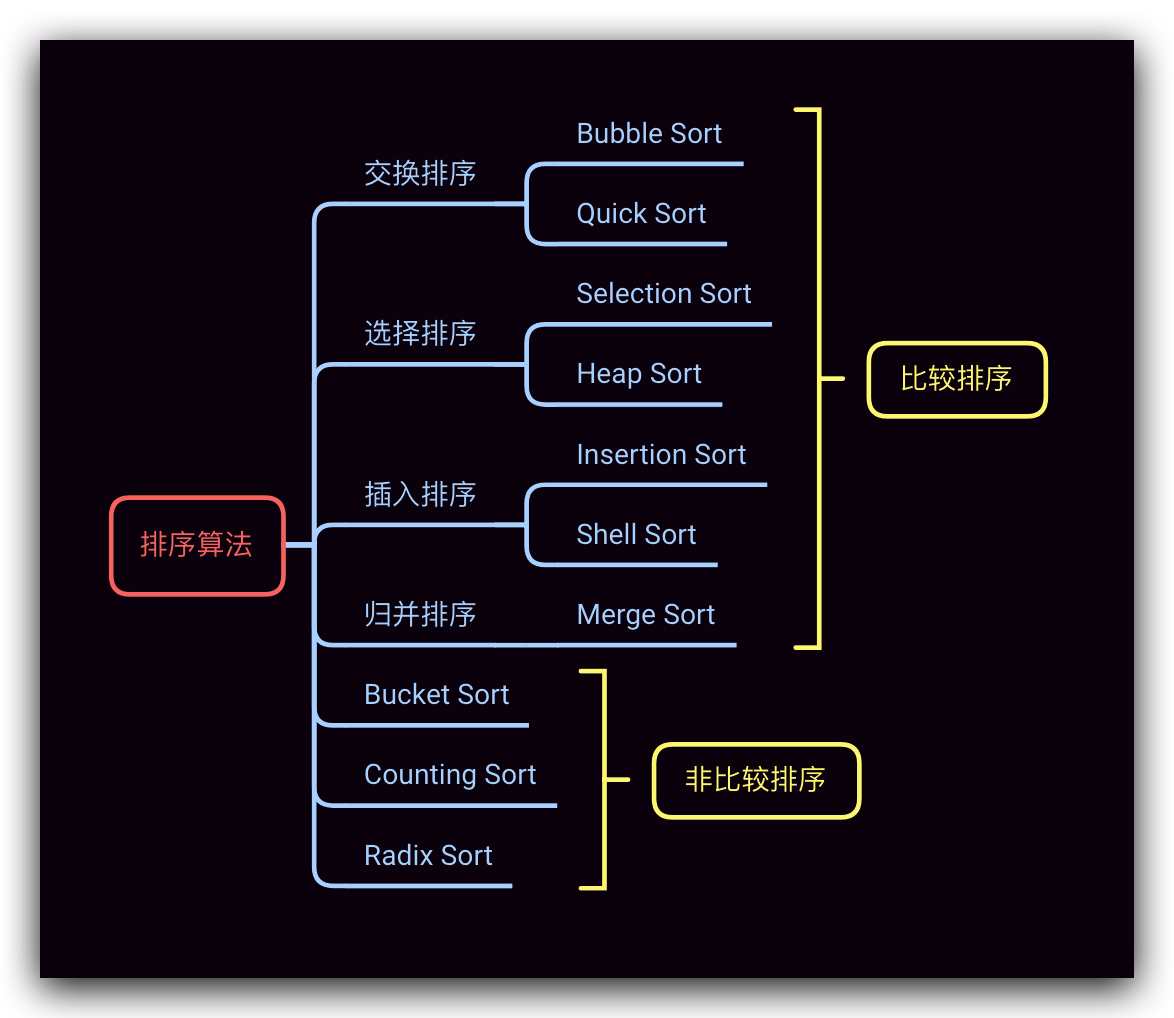# 切分过程详解

## 切分目的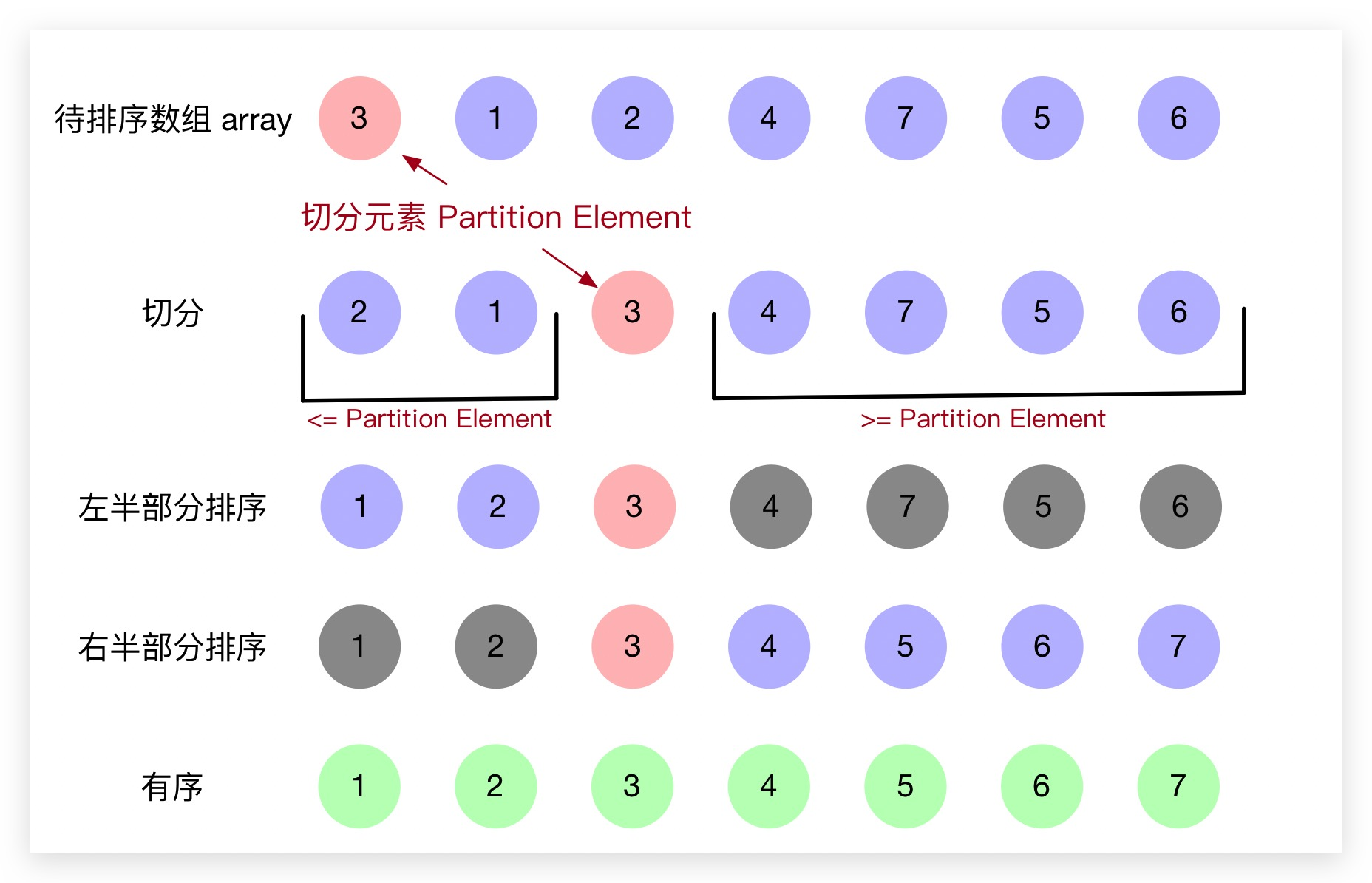## 切分原理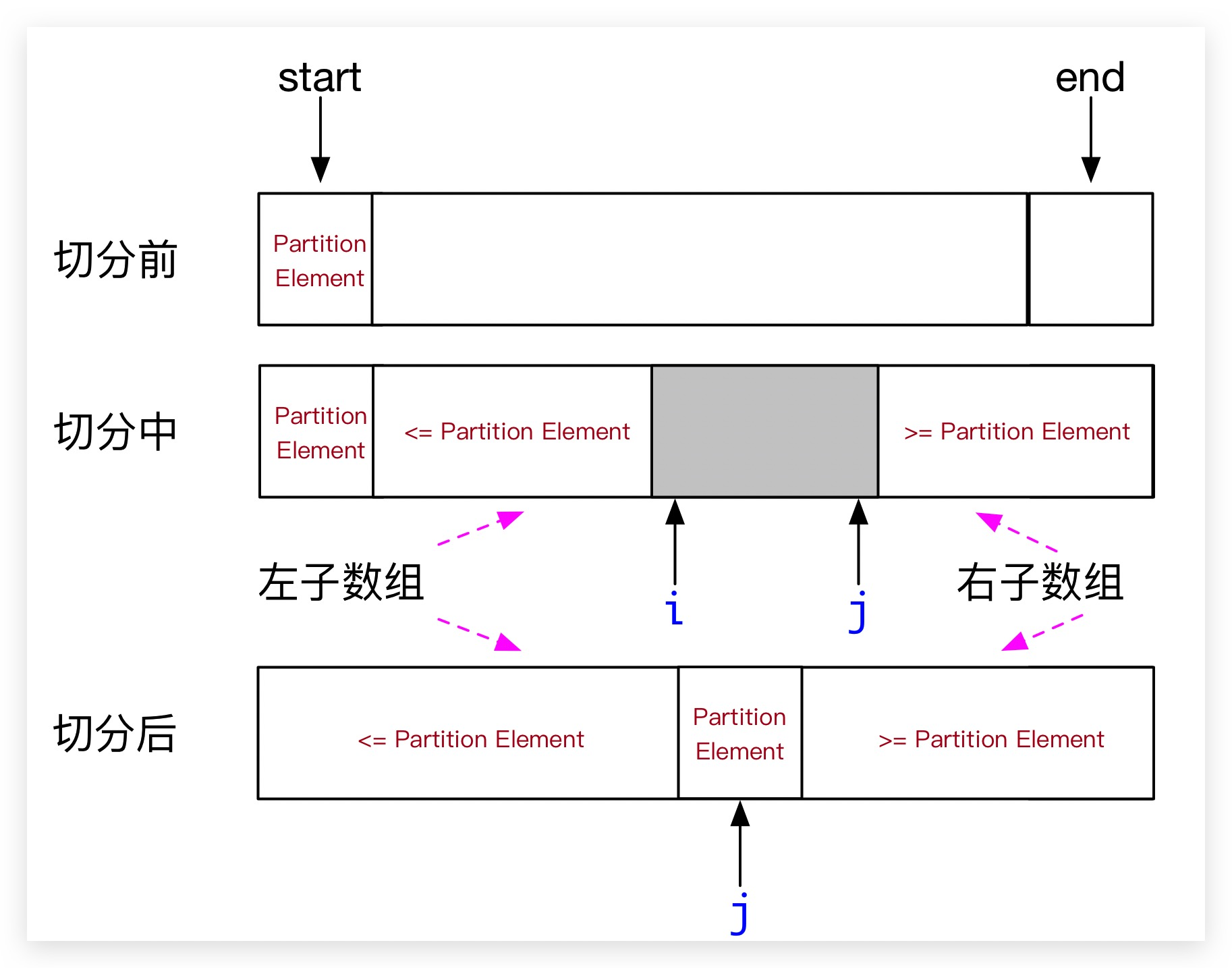## Java 实现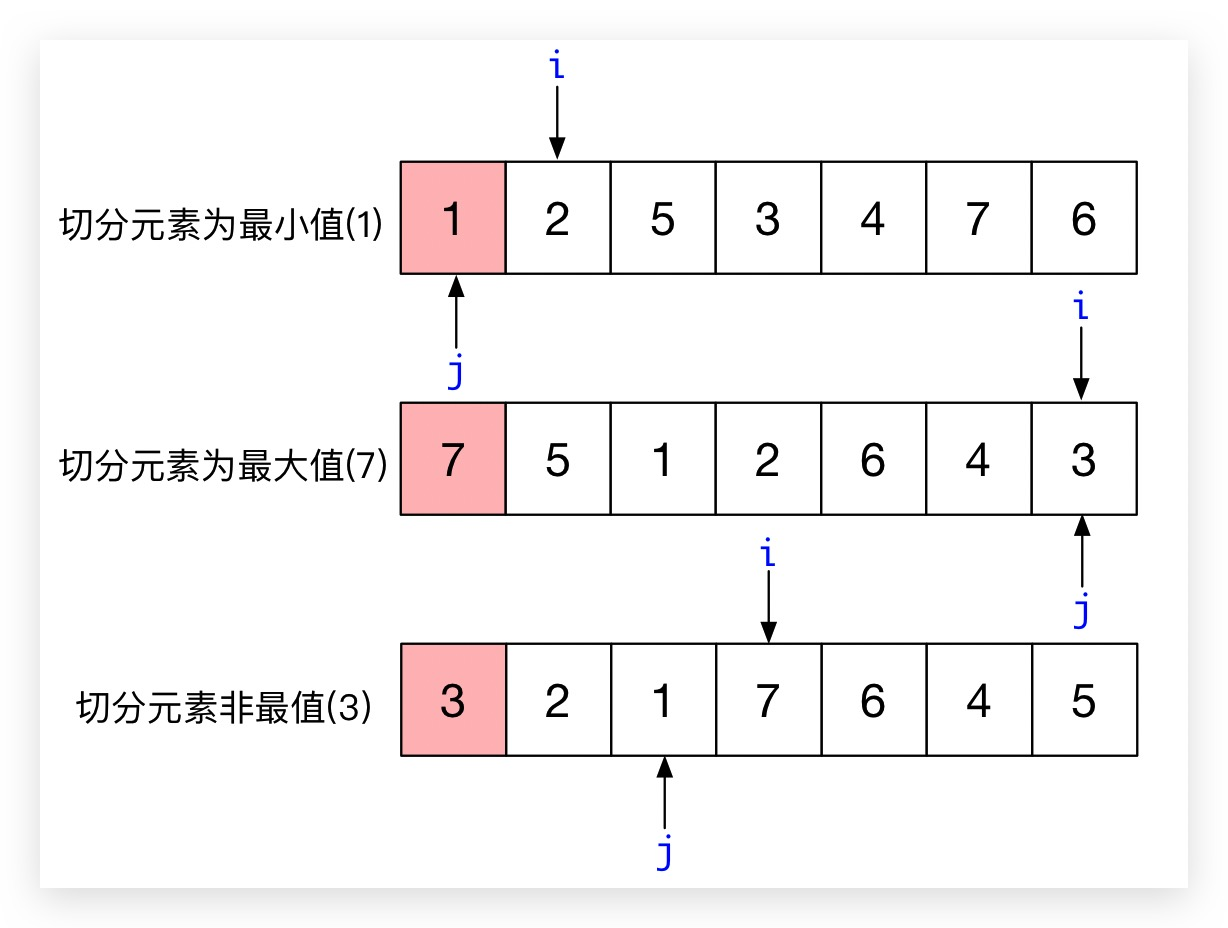# 基础快速排序

## Java 实现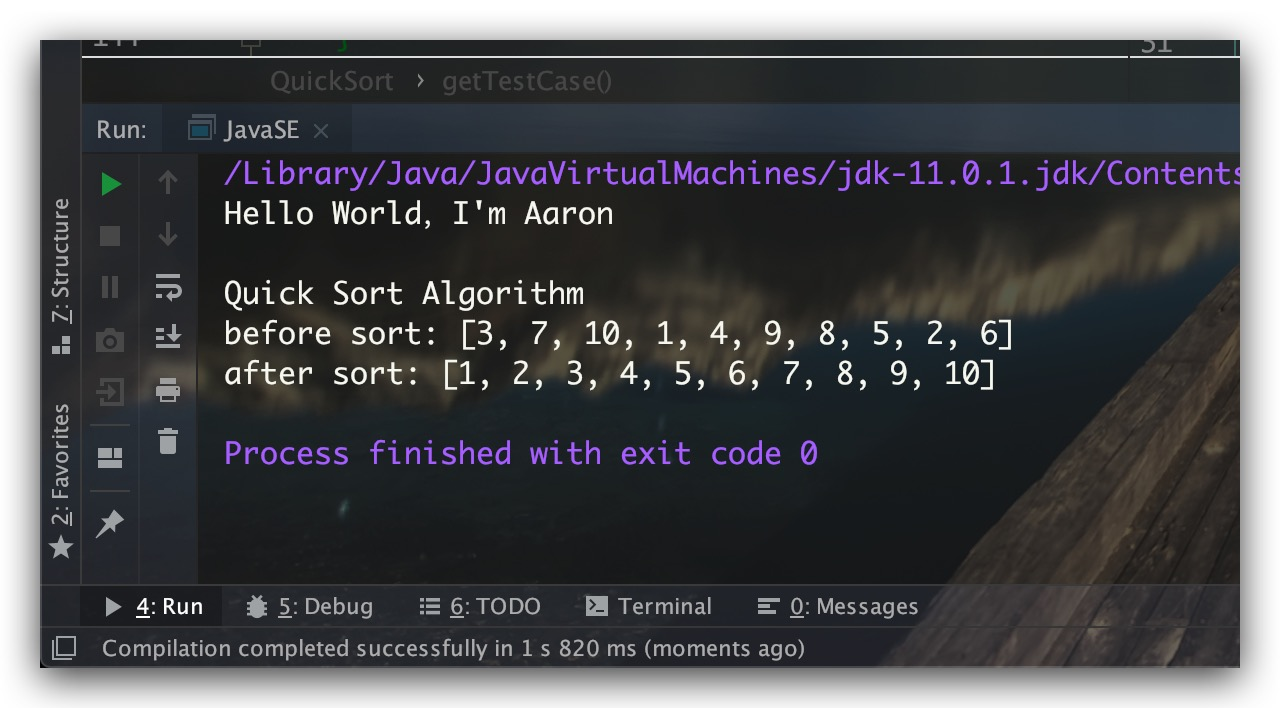## 特点

$O(N \log N)$ $O(N^2)$ $O(N \log N)$ $O(\log N)$ 不稳定

• 保证随机性 : 排序前先将元素打乱；每次任意地选取不同位置上的元素作为切分元素
• 处理与切分元素相等的元素情况 : 在上文的”扫描-交换”过程，左游标是遇到一个大于等于切分元素的元素停下；右游标是遇到一个小于等于切分元素的元素停下。虽然这样处理可能会导致一些不必要的元素交换，但可以一定程度上避免切分点过于靠近数组的两端

# 基于三向切分的快速排序

## 原理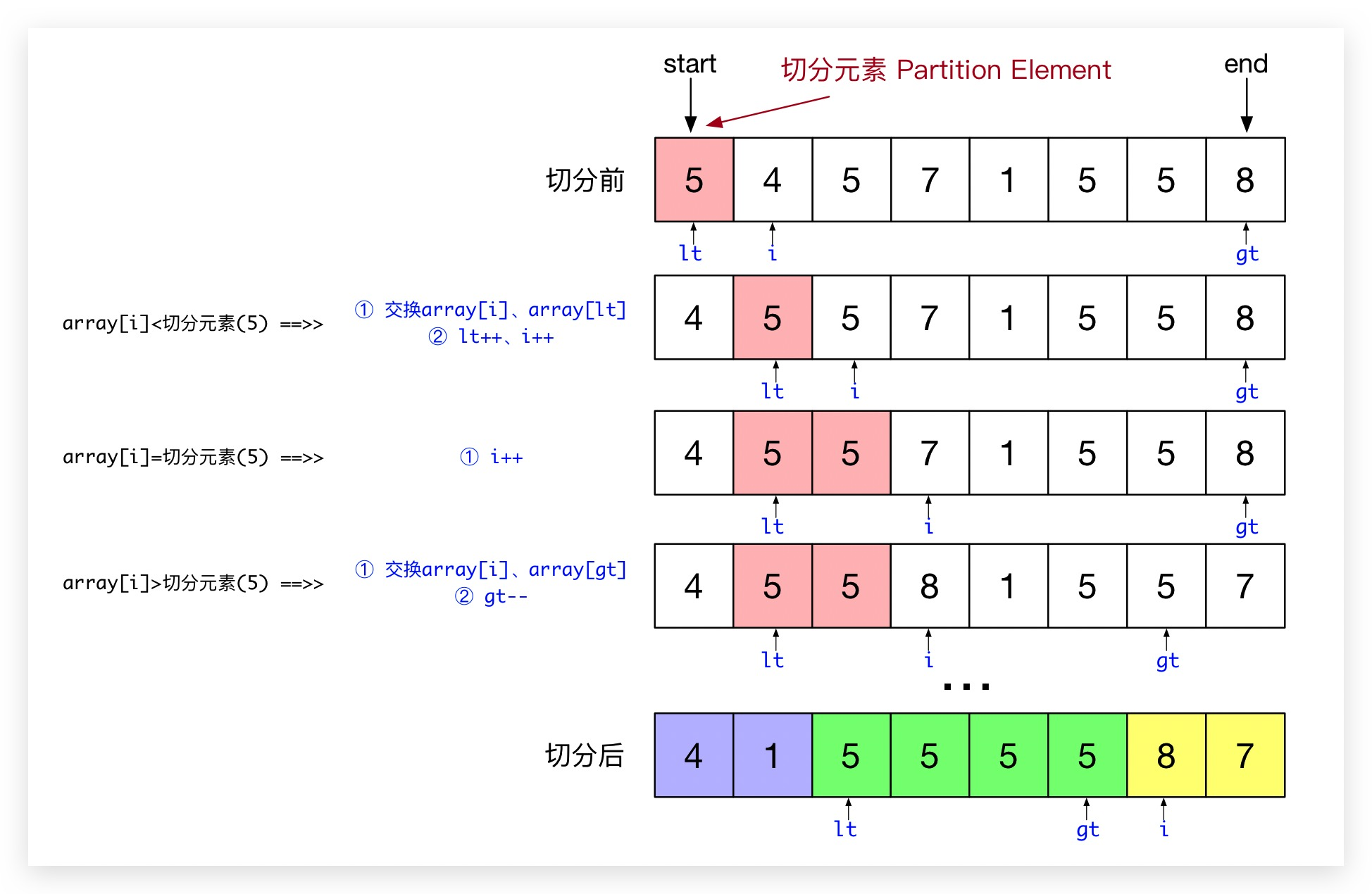## Java 实现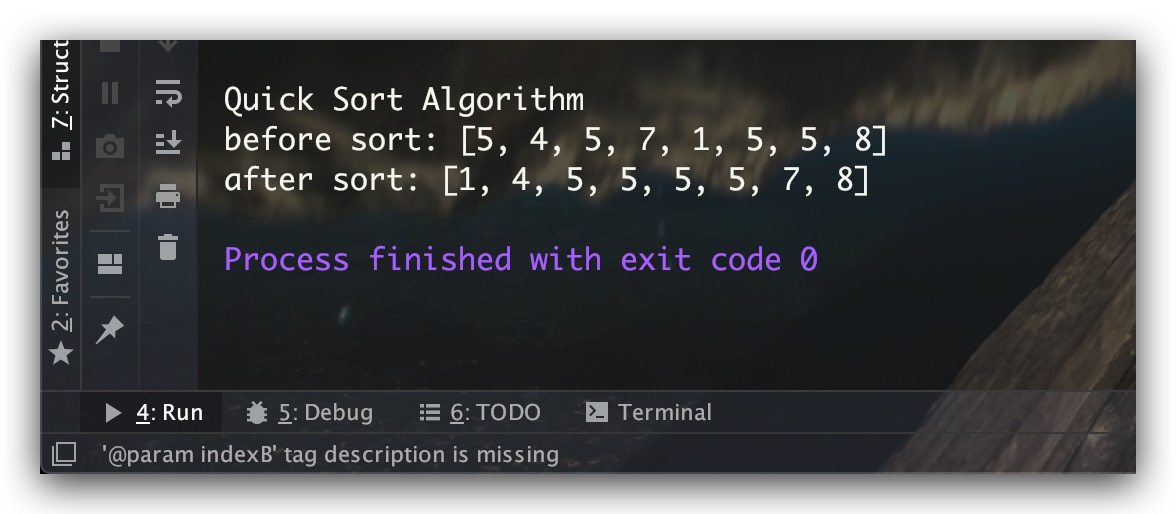## 特点

$O(N \log N)$ $O(N^2)$ $O(N)$ $O(\log N)$ 不稳定

# 参考文献

1. 算法(第4版) Robert Sedgewick、Kevin Wayne 著
0%